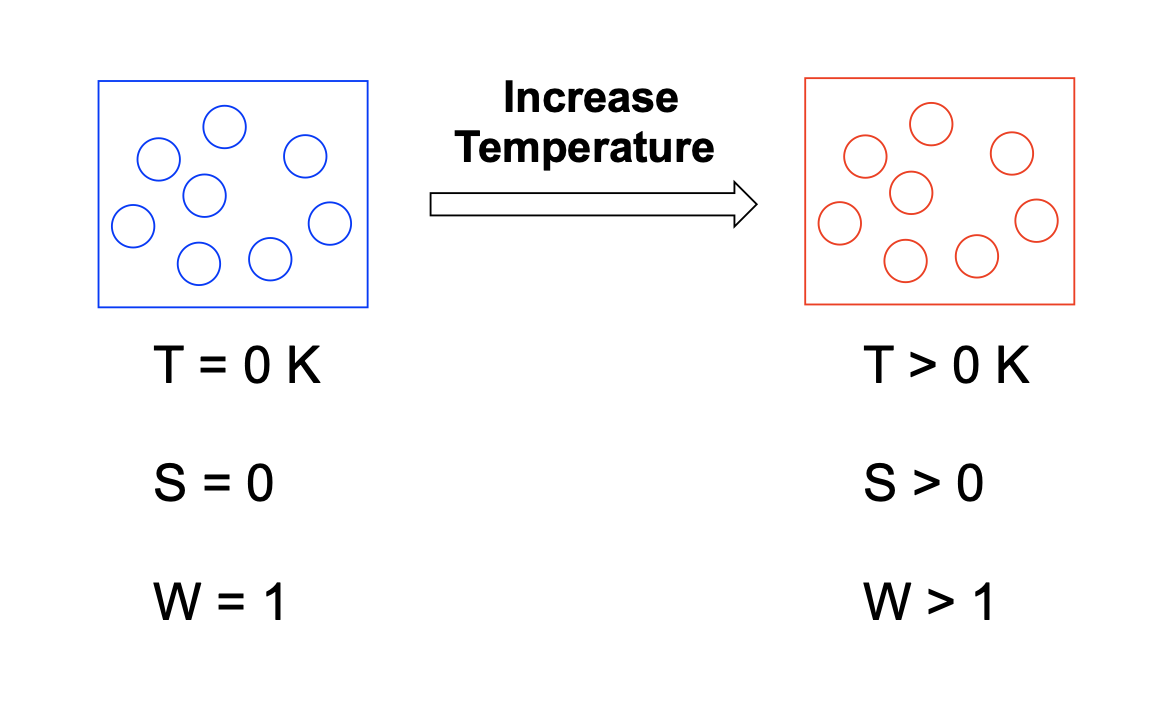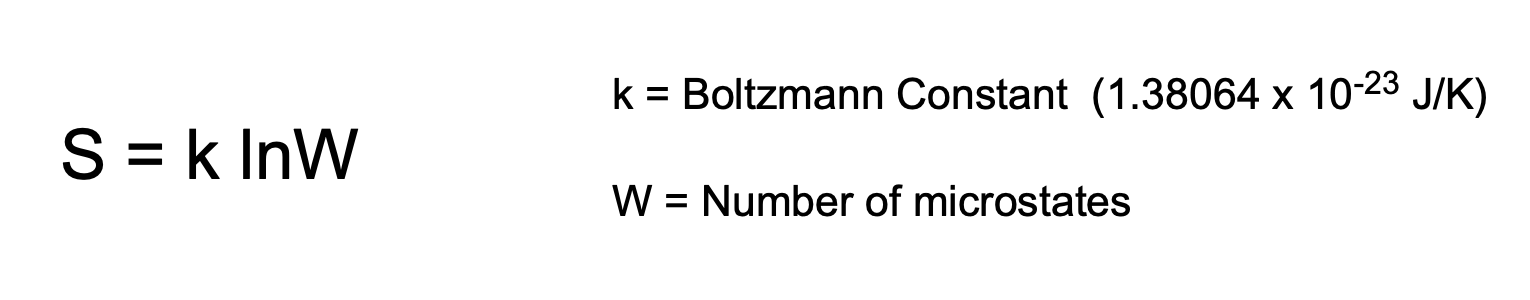Clutch Prep is now a part of Pearson
Ch.17 - Chemical ThermodynamicsWorksheetSee all chapters

# Third Law of Thermodynamics

See all sections
Sections
Spontaneous vs Nonspontaneous Reactions
Entropy
Entropy Calculations
Entropy Calculations: Phase Changes
Third Law of Thermodynamics
Gibbs Free Energy
Gibbs Free Energy Calculations
Gibbs Free Energy And Equilibrium

The Third Law of Thermodynamics examines the state of entropy for a given substance at absolute zero.

###### Absolute Zero & Entropy

Concept #1: The entropy of a closed system can be related to its temperature.

Generally as the temperature of the system increases there will be an increase in the number of microstates and therefore an increase in entropy.Example #1: All the statements are correct except:

a) greater number of molecular motion, greater number of possible microstates

b) a perfectly ordered system has more than 1 microstate

c) any system at a temperature above 0 K has a positive ∆S

d) perfect crystal exhibits no molecular motion

Concept #2: The Boltzmann Equation allows for the calculation of entropy by examining the different energetic configurations of a compound.Example #2: Consider a system with a total of 3 x 1026 number of microstates, what is the entropy of such a system?

Practice: A brand new deck of cards which hasn’t been shuffled yet, possesses only one arrangement. Another, older deck has been shuffled and possesses 8 × 1067 arrangements. Calculate and compare entropies of each deck.